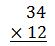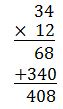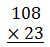# How to Multiply Large Numbers: Steps and Examples

An error occurred trying to load this video.

Try refreshing the page, or contact customer support.

Coming up next: How to Perform Division: Steps & Examples

### You're on a roll. Keep up the good work!

Replay
Your next lesson will play in 10 seconds
• 0:01 Multiplying Large Numbers
• 0:58 The Procedure
• 1:48 Example 1
• 4:14 Example 2
• 6:29 Lesson Summary
Save Save

Want to watch this again later?

Timeline
Autoplay
Autoplay
Speed Speed Audio mode

#### Recommended Lessons and Courses for You

Lesson Transcript
Instructor: Yuanxin (Amy) Yang Alcocer

Amy has a master's degree in secondary education and has taught math at a public charter high school.

After watching this video lesson you will be able to multiply any two numbers together. You will learn how to use your knowledge of multiplying smaller numbers together to multiply larger numbers with ease.

## Multiplying Large Numbers

Okay, so you know about multiplication: that it means to add a number a certain amount of times. You've memorized your multiplication table and you can multiply two small numbers together with ease. For instance, if you were given the problem 3 * 5, you would immediately realize that it means that you are going to add the 3 five times. You might have pictured 5 groups of 3 donuts that you needed to add up. So, you would do 3 + 3 + 3 + 3 + 3. What does this all equal? It equals 15.

And since you've memorized your multiplication table, you didn't need to do all that addition. You just knew it! But what if you need to perform long multiplication, to multiply two larger numbers together, such as 12 * 34? What would you do then? Well, keep watching this video lesson and you will learn a procedure you can use to do just that.

## The Procedure

So, let me explain the procedure to you first before showing you. What you will need to know in order to use this procedure is just your knowledge of your basic multiplication, your multiplication skills of small numbers. So, the way this procedure works is you first write your two numbers one on top of the other. And then you begin multiplying the digits by themselves.

You begin by multiplying the last digit of your bottom with your digits of your top number starting from the right and working your way to the left. After you are done multiplying all the digits of your top number, you move on to the next digit of your bottom number. You'll move from right to the left. So now, let me go through an example with you in more detail. This way, you will be able to see what is going on.

## Example 1

So, let's multiply 12 with 34. We first write our numbers one on top of the other. Since we are multiplying, it doesn't matter which number we write on top. It's usually easier to write your smaller number on the bottom. So, we will do just that. We will write 34 on top and 12 on the bottom. We draw a line underneath the last number and put an 'x' to the left of our bottom number to let us know that we are multiplying.Now, we start our multiplication of our numbers digit by digit. We always move right to left. We start by multiplying the last digit of our bottom number with the digits of our top number, moving from right to left. So, our first multiplication is 2 * 4. It equals 8. We write this 8 underneath the 2. We are moving right to left, so our next multiplication is 2 * 3. What does this equal? It equals 6, so I write the 6 to the left of my 8. So, my first line of my figuring out my answer part is 68.

Now, I'm going to move to the next digit of my bottom number. I am moving right to left, so the digit to the left of the 2 is 1. So, I multiply this number with the digits of my top number, moving right to left. I'm basically repeating what I did with the 2. I multiply my 1 with the 4 first. What is 1 * 4? It is 4. But instead of writing this underneath the 2, I now move one space to the left and write it under the 1. I can put a 0 in line with the 2 to remind me that I don't need a number there.

Now, I've already written something in the space that is directly under the 1, so I write it under that number in line with the 1. Next, I multiply the 1 with the 3. 1 * 3 is 3, so I write this to the left of the 4 to continue my answer for this part. Now that I've multiplied everything together, I draw a line underneath my last line. I am going to add now to find my final answer, so I put a plus sign to the left of my last number. I add 68 + 340. It equals 408. I am now done, and 408 is my answer.## Example 2

Let's try another one. Let's do 108 * 23. I set up my problem like before. I write 108 on top and 23 on bottom because 23 is the smaller number. I draw a line underneath and put an 'x' to signify that I will be doing multiplication.To unlock this lesson you must be a Study.com Member.

### Register to view this lesson

Are you a student or a teacher?

#### See for yourself why 30 million people use Study.com

##### Become a Study.com member and start learning now.
Back
What teachers are saying about Study.com

### Earning College Credit

Did you know… We have over 220 college courses that prepare you to earn credit by exam that is accepted by over 1,500 colleges and universities. You can test out of the first two years of college and save thousands off your degree. Anyone can earn credit-by-exam regardless of age or education level.